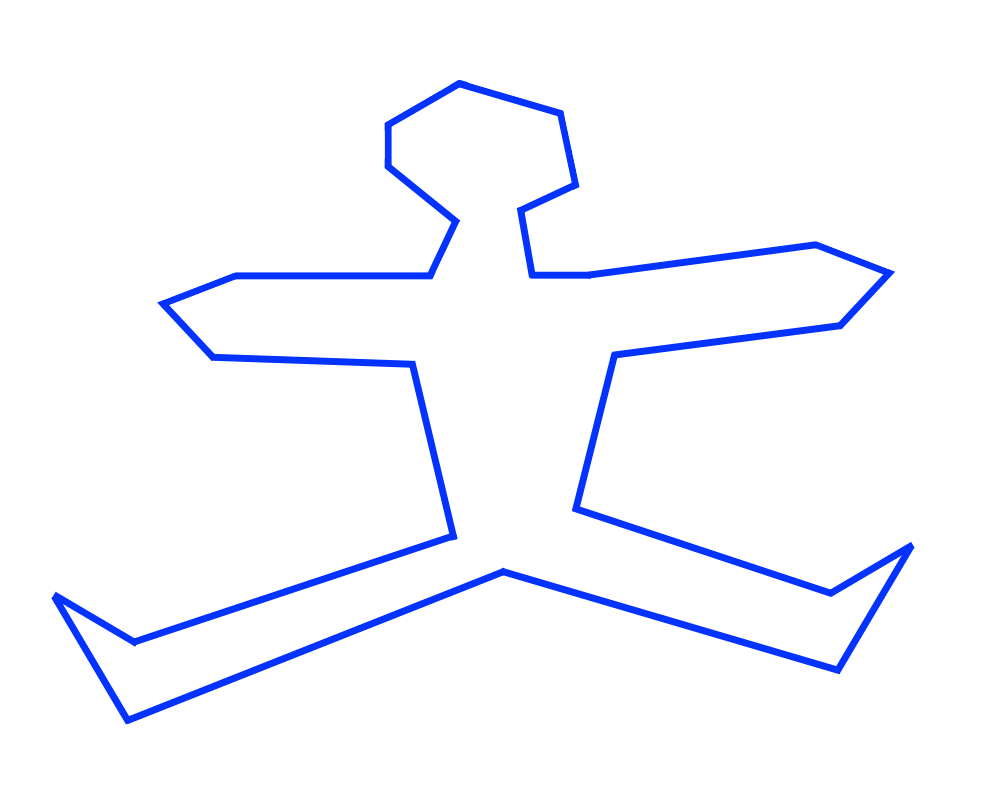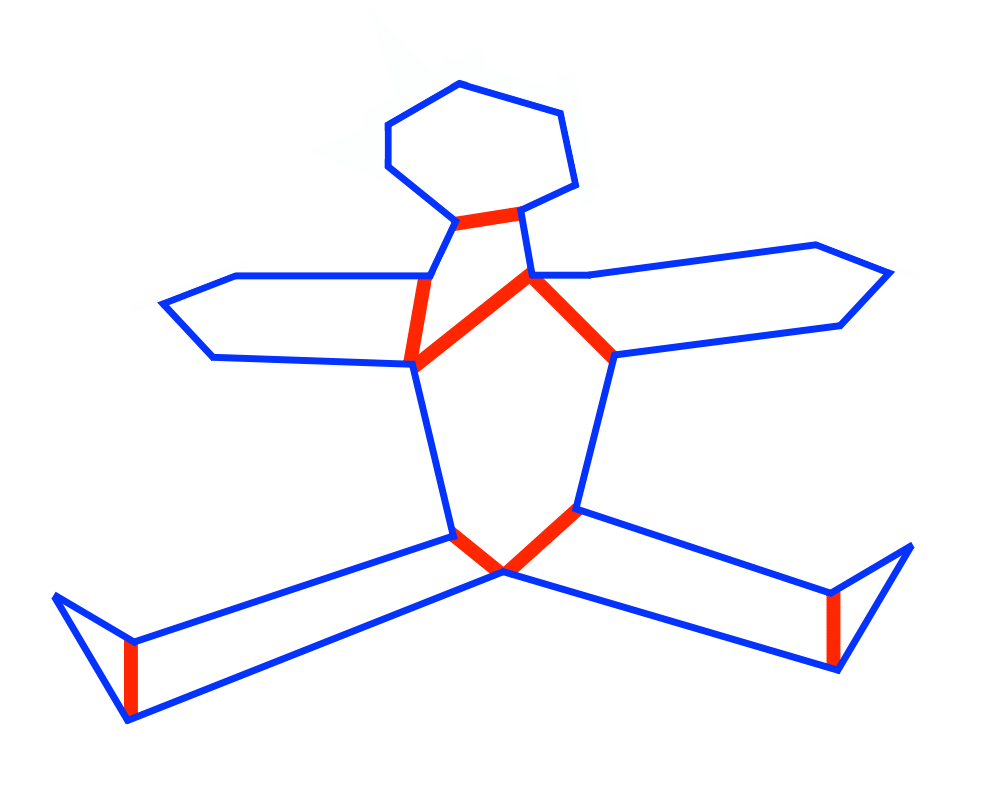# Polygon PartitioningInputOutput

Input Description: A polygon or polyhedron $$P$$.
Problem: How can $$P$$ be partitioned into a small number of simple (typically convex) pieces?

Excerpt from The Algorithm Design Manual: Polygon partitioning is an important preprocessing step for many geometric algorithms, because most geometric problems are simpler and faster on convex objects than on nonconvex ones. We are better off whenever we can partition a nonconvex object into a small number of convex pieces, because it is easier to work with the pieces independently than with the original object.

Go To Main Page